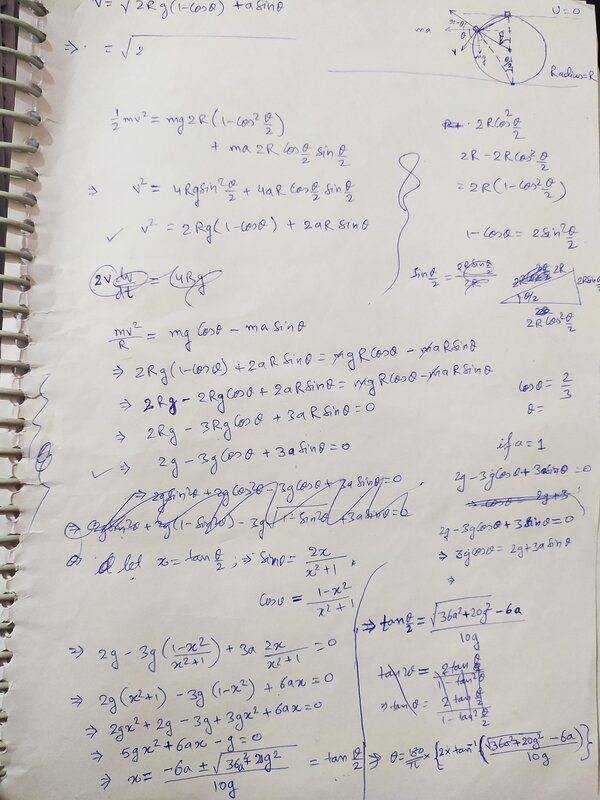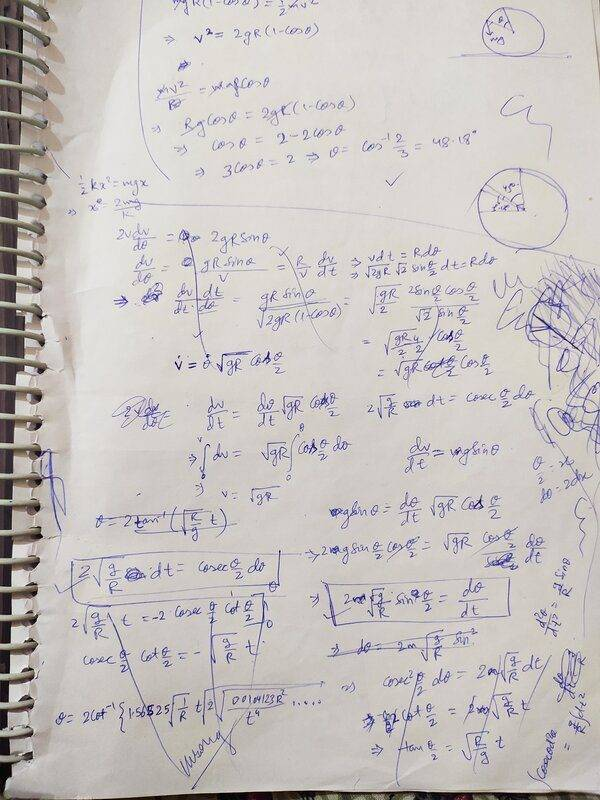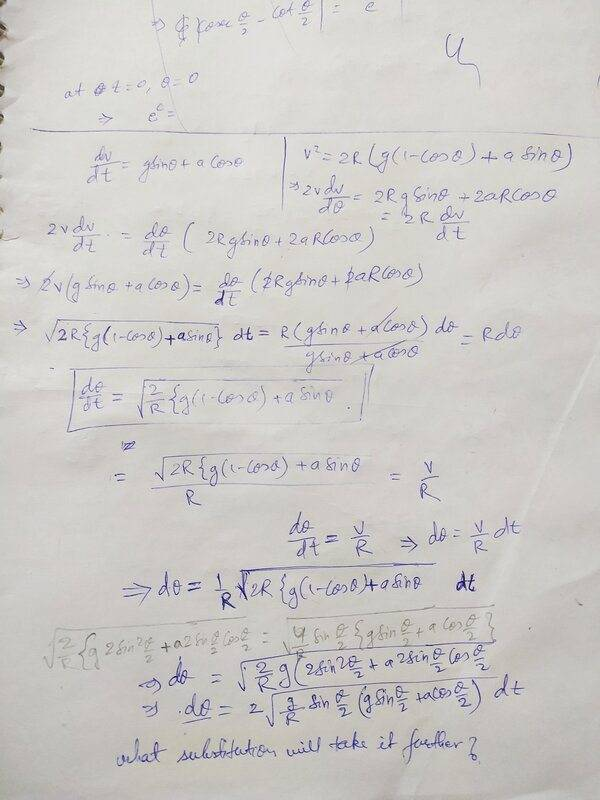# What substitution/other method to use to solve this differential equation?

• Amit1011
In summary, the conversation discusses a differential equation involving a smooth sphere accelerating horizontally and a particle released at an angle on the surface of the sphere. The equation is solved up to a certain point, but the solution cannot be found analytically. It is suggested that the problem may need to be solved numerically. A previous related thread is referenced and it is mentioned that the problem can be solved with a substitution. The possibility of an analytic solution is also mentioned.f

#### Amit1011

Homework Statement
If a particle is almost at the top of a smooth sphere, but not exactly at the very top, and then starts to slide, and the particle also experiences a constant horizontal acceleration(a m/sec^2) in addition to acceleration due to gravity, then what will be the equation between theta (the angle it slides from its initial position) and time, until it flies off the sphere ?
Relevant Equations
From Energy conservation: $$\frac{1}{2}mv^{2}=mgR(1-cos\theta )+maRsin\theta$$
From Newton's laws of Motion: $$m\frac{dv}{dt}= mgsin\theta +macos\theta$$
Differentiating eq1 mentioned above, and using eq 2, i got : $$v\frac{dv}{d\theta}=R\frac{dv}{dt}$$
From this, i got:$$\frac{d\theta}{dt}=\sqrt{(2/R)(g(1-cos\theta )+asin\theta)}$$

After this point, I am not able to understand what substitution or may be other method could be used to solve this differential equation. Its more of a math problem at this point, instead of a physics problem. If possible, let me know how to solve this differential equation. Any help would be welcome.

Where is the horizontal force of magnitude ##ma##, derived from the potential energy ##-maR\sin{\theta}##, coming from? Is the sphere accelerating horizontally, and ##ma## is the magnitude of the fictitious force that appears in the non-inertial frame? Or is it something else entirely?

•Amit1011
Where is the horizontal force of magnitude ##ma##, derived from the potential energy ##-maR\sin{\theta}##, coming from? Is the sphere accelerating horizontally, and ##ma## is the magnitude of the fictitious force that appears in the non-inertial frame? Or is it something else entirely?
Yes, it is exactly how you described it. But I thought about it; figured that if the particle has some charge on it and it is in an Electric field, then also, it will experience a force whose magnitude could be ma. But what you assumed about the frame of reference being non-inertial is how the initial condition is given.

OK, great. If the force were instead of electric origin, or something, then I probably wouldn't write it as "##ma##" if ##a## is the horizontal acceleration that would result if the electric force were applied in isolation, but that's besides the point. So would I be right in saying that the problem statement is:

"A smooth sphere accelerates horizontally to the left with acceleration of magnitude ##a##, and a particle is released from a point on the surface of the sphere at an angle ##\theta_0## to the right of the vertical passing through the centre of the sphere, such that it is initially at rest in the frame of the sphere. During the interval in which the particle is in contact with the sphere, find ##\theta(t)##".

I can't see how to solve that differential equation analytically, but perhaps someone else will be able to help.

•Amit1011
OK, great. If the force were instead of electric origin, or something, then I probably wouldn't write it as "##ma##" if ##a## is the horizontal acceleration that would result if the electric force were applied in isolation, but that's besides the point. So would I be right in saying that the problem statement is:

"A smooth sphere accelerates horizontally to the left with acceleration of magnitude ##a##, and a particle is released from a point on the surface of the sphere at an angle ##\theta_0## to the right of the vertical passing through the centre of the sphere, such that it is initially at rest in the frame of the sphere. During the interval in which the particle is in contact with the sphere, find ##\frac{d\theta}{dt}##".

I can't see how to solve that differential equation analytically, but perhaps someone else will be able to help.

Yes, you've put it quite nicely; only in the last line, it should have been: find $$\theta =f(t)$$

•etotheipi
Whoops, you're quite right, that's a lack of caffeine speaking. I've corrected it now•Amit1011
I tried to solve the differential equation by paper pen.. couldn't make it work. I then tried wolfram alpha, it just showed various forms of the same equation, but not the solution. Is there any way that this differential equation could be solved ? If somebody knows, kindly let me know.

I suspect that there is no analytic solution and it will need to be solved numerically.

I tried to solve the differential equation by paper pen.. couldn't make it work. I then tried wolfram alpha, it just showed various forms of the same equation, but not the solution. Is there any way that this differential equation could be solved ? If somebody knows, kindly let me know.
First you solve the differential equation with ##a=0## as you started doing in the thread
https://www.physicsforums.com/threa...weird-equation-between-angle-and-time.992749/
that was closed for moderation and has now been reopened. I gave you the substitution in post #27. Please try harder.

Once you understand how that problem is solved, this new problem is the same as that one except that the constant acceleration is in a different direction and has different magnitude. The adjustment should be easy to make.

Last edited:
I suspect that there is no analytic solution and it will need to be solved numerically.
I believe there is. See post #9.

•phyzguy
First you solve the differential equation with ##a=0## as you started doing in the thread
https://www.physicsforums.com/threa...weird-equation-between-angle-and-time.992749/
that was closed for moderation and has now been reopened. I gave you the substitution in post #27. Please try harder.

Once you understand how that problem is solved, this new problem is the same as that one except that the constant acceleration is in a different direction and has different magnitude. The adjustment should be easy to make.

Substituting ## 1-cos\theta = 2sin^2\frac{\theta }{2} ## is the first thing I tried when I was doing it on paper; however, couldn't make a dent. I understand that putting a=0, reduces it to the simpler version of the differential equation. But the present differential equation is not getting solved. I also understand that the graph will be similar in looks but much steeper in present case than it was when a was =0.

Substituting is the first thing I tried when I was doing it on paper; however, couldn't make a dent

No need to Shout.. !

For a moment there, felt like I am sitting in a class getting shouted on by an Angry teacher who thinks that the student asking the question has not done his due.
Let me post the pages on which I've been working to prove that wrong.

For a moment there, felt like I am sitting in a class getting shouted on by an Angry teacher who thinks that the student asking the question has not done his due.
I felt like that teacher except I was not angry and I was not shouting. The convention is that capital letters is shouting, lower case is not. I used large letters to get your attention to the fact that you need to show what you have done. So far all I have seen from you is that you tried this or that equation solver and it didn't work. What is the logical conclusion in view of the fact that I gave you the substitution to help you proceed on your own and without the need of an equation solver?

After this point, I am not able to understand what substitution or may be other method could be used to solve this differential equation.
Hi Amit:

Your last equation in post #1 has the form
dθ/dt = f(θ) .​
Have you tried to work with its reciprocal:
dt = dθ/f(θ)?​

Regards,
Buzz

I felt like that teacher except I was not angry and I was not shouting. The convention is that capital letters is shouting, lower case is not. I used large letters to get your attention to the fact that you need to show what you have done. So far all I have seen from you is that you tried this or that equation solver and it didn't work. What is the logical conclusion in view of the fact that I gave you the substitution to help you proceed on your own and without the need of an equation solver?These are the all pages on which I made attempt to solve this question from start to finish, apparently I couldn't finish it. Most of it will seem like illegible on first sight, but it isn't illegible to me. On the 3rd page, at the bottom, where I had written with pencil first, but then felt like it wouldn't be legible as I was taking pic where lighting condition were not proper, I rewrote the last 2 lines with pen. After I figured the differential equation that I had also posted in the beginning of the topic, I made the substitution ##1-cos\theta = 2sin^2\frac{\theta }{2}##, but after that, still couldn't make it work further. That's when I posted it on this forum with the title" What substitution/other method to use to solve this differential equation?" I was only interested in the substitution that might have worked.

You can make all the judgement in the world about my capacity/incapacity to make it work after substituting ##1-cos\theta = 2sin^2\frac{\theta }{2}##, but that's what i honestly put in the description of the post that, at this point it is more of a math problem instead of being a physics problem. I also wrote in comment # 11, that I tried substituting, but couldn't make a dent. That didn't mean that a dent couldn't be made by someone else.

Thank you @Buzz Bloom for your kind suggestion. Will try to see if your suggestion works.

However, in just 2 days of my being on this forum, I have realized that this forum is not for me. So no point in writing any further.

However, in just 2 days of my being on this forum, I have realized that this forum is not for me. So no point in writing any further.
Set the forum aside for a moment and think if physics is for you. If it is, there is a point in writing further. I will try one more time to show you what I expected. You have the equation$$\frac{d\theta}{dt}=\sqrt{\frac{2g}{R}(1-\cos\theta)}.$$
You make the substitution to get$$\frac{d\theta}{dt}=\sqrt{\frac{2g}{R}2\sin^2(\theta/2)}.$$What do you think comes next? Hint: separate variables.

•